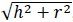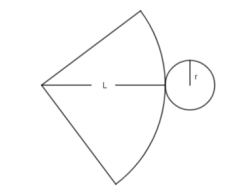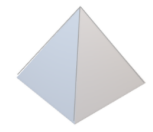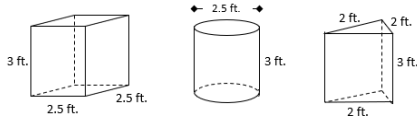# MA.912.GR.4.6Export Print
Solve mathematical and real-world problems involving the surface area of three-dimensional figures limited to cylinders, pyramids, prisms, cones and spheres.
General Information
Subject Area: Mathematics (B.E.S.T.)
Strand: Geometric Reasoning
Status: State Board Approved

## Benchmark Instructional Guide

• Circle
• Cone
• Cylinder
• Prism
• Pyramid
• Rectangle
• Sphere
• Square
• Triangle

### Vertical Alignment

Previous Benchmarks

Next Benchmarks

### Purpose and Instructional Strategies

In middle grades, students determined surface area using nets and formulas for right rectangular prisms, right rectangular pyramids and right circular cylinders. In Geometry, students explore for the first time the surface area of cones and spheres. Instruction includes reviewing units and conversions within and across different measurement systems (as this was done in middle grades).
• Instruction includes discussing the convenience of answering with exact values (e.g., the simplest radical form or in terms of pi) or with approximations (e.g., rounding to the 22 nearest tenth or hundredth or using 3.14, $\frac{\text{22}}{\text{7}}$ or other approximations for pi). It is also important to explore the consequences of rounding partial answers on the accuracy or precision of the final answer, especially when working in real-world contexts.
• Instruction includes reviewing the definition of cylinders, pyramids, prisms, cones and spheres (as this was done in grade 5), and discussing the definitions of right and oblique polyhedrons, cubes, tetrahedrons, regular prisms and regular pyramids.
• Instruction includes the connection to finding areas of two-dimensional figures to determine the surface area of cylinders, pyramids, prisms and cones.
• For example, the surface area of a cylinder is the result of combining the area of the bases (circles with radius $r$) with the lateral area (a rectangle with base $C$ = 2π$r$ and height equal to the height of the cylinder). The area of the circles is π$r$and the area of the rectangle is $b$$h$ which is equivalent to $C$$h$ which is equivalent to 2 π$r$$h$. Therefore, given a cylinder with radius $r$ and height $h$, its surface area is 2π$r$2 + 2π$r$$h$
• For example, the surface area of a cone is the result of combining the area of the base (a circle with radius $r$ and circumference $C$) and the area of the curved surface (a circular sector with radius $L$, which is the slant height of the cone and arc length $C$). The slant height $L$ is, where $h$ is the height of the cone. The area of the circle is π$r$2 and the area of the circular sector is $\frac{\text{1}}{\text{2}}$ (arc length)(radius), which is equivalent to $\frac{\text{1}}{\text{2}}$$C$$L$, which is equivalent to $\frac{\text{1}}{\text{2}}$(2π$r$)$L$, which is equivalent to πrL. Therefore, given a cone with radius $r$ and height $h$, its surface area if  π$r$2 +  π$r$$L$ = π$r$2 + π$r$is π$r$($r$ +).• Instruction includes exploring the surface area of cylinders, pyramids and prisms as the result of combining areas of triangles, rectangles and circles (and when needed, other polygons). Students should understand the similarities and differences between lateral area and surface area. (MTR.2.1)
• Since deriving the surface area of a sphere requires Calculus, students will not be able to explore its formula and can be calculated using the formula $S$$A$ = 4π$r$2
• Instruction includes exploring a variety of real-world situations where finding the surface area is relevant for different purposes. Problem types include components like percentages, cost and budget, constraints, comparisons, or others. Problem types include finding missing dimensions given the surface area of a three-dimensional figure, finding the surface area of composite figures or determining which face to include in calculations within real-world context (i.e., the surface area required to paint a house, the surface area that will be covered by a label in a soup can).

### Common Misconceptions or Errors

• Students may have trouble working with formulas by making incorrect substitutions or incorrect use of the order of operations.

• There are three Pyramids of Giza. The largest, the Great Pyramid, has an approximately square base with side lengths averaging 230 meters and a lateral surface area of 85,836 square meters. What is the height of the Great Pyramid?• The surface area of a sphere with radius 10 is 400π square units.
• Part A. Discuss the value of this kind of answer for its accuracy and precision.
• Part B. Discuss the effect of replacing π in the formulas with 3.14, 3.1416, $\frac{\text{22}}{\text{7}}$ and other approximations. What happens with the answer, the surface area of the figure, in each case?

### Instructional Items

Instructional Item 1
• Kristin and Rachel are hosting an art show where they will showcase local artists’ sculptures. They are painting pedestals upon which the sculptures will be placed. Pictures of the pedestals they will be using are below. One gallon of paint can cover 400 square feet.• Part A. How many gallons of paint will they need to purchase to cover at least 4 of each type of pedestal? Assume that the base of each will not be painted.
• Part B. If there is any paint left over, determine how many of which shape pedestals could be painted.

*The strategies, tasks and items included in the B1G-M are examples and should not be considered comprehensive.

## Related Courses

This benchmark is part of these courses.
1200400: Foundational Skills in Mathematics 9-12 (Specifically in versions: 2014 - 2015, 2015 - 2022, 2022 and beyond (current))
1206310: Geometry (Specifically in versions: 2014 - 2015, 2015 - 2022, 2022 and beyond (current))
1206320: Geometry Honors (Specifically in versions: 2014 - 2015, 2015 - 2022, 2022 and beyond (current))
7912070: Access Mathematics for Liberal Arts (Specifically in versions: 2014 - 2015, 2015 - 2018, 2018 - 2019, 2019 - 2022, 2022 - 2023, 2023 and beyond (current))
1206315: Geometry for Credit Recovery (Specifically in versions: 2014 - 2015, 2015 - 2022, 2022 and beyond (current))
7912065: Access Geometry (Specifically in versions: 2015 - 2022, 2022 and beyond (current))
1207350: Mathematics for College Liberal Arts (Specifically in versions: 2022 and beyond (current))

## Related Access Points

Alternate version of this benchmark for students with significant cognitive disabilities.
MA.912.GR.4.AP.6: Solve mathematical or real-world problems involving the surface area of three-dimensional figures limited to cylinders, pyramids, prisms, and cones.

## Related Resources

Vetted resources educators can use to teach the concepts and skills in this benchmark.

## Formative Assessments

Windy Pyramid:

Students are asked to use a net to find the surface area of a triangular pyramid.

Type: Formative Assessment

Skateboard Ramp:

Students are asked to draw a net of a three-dimensional figure.

Type: Formative Assessment

Prismatic Surface Area:

Students are asked to determine the surface area of a right triangular prism and explain the procedure.

Type: Formative Assessment

## Lesson Plans

Three Dimensions Unfolded:

Students will use nets of prisms to find the surface area of composite 3-D figures. Students will learn to identify the faces of 3-D figures that are needed to find the surface areas, and those that are not needed.

Type: Lesson Plan

Wrapping Up Geometry (Surface Area of Triangular Prisms) :

This lesson is designed to take students from recognizing nets of triangular prisms and finding areas of their individual faces, to finding the surface area of triangular prisms.

Type: Lesson Plan

In this activity, students will utilize measurement data provided in a chart to calculate areas, volumes, and densities of cookies. They will then analyze their data and determine how these values can be used to market a fictitious brand of chocolate chip cookie. Finally, they will integrate cost and taste into their analyses and generate a marketing campaign for a cookie brand of their choosing based upon a set sample data which has been provided to them.

Type: Lesson Plan

Turning Tires Model Eliciting Activity:

The Turning Tires MEA provides students with an engineering problem in which they must work as a team to design a procedure to select the best tire material for certain situations. The main focus of the MEA is applying surface area concepts and algebra through modeling.

Type: Lesson Plan

## Perspectives Video: Expert

Implications of the Spherical Earth:

To understand atmospheric and oceanic currents, one needs a well-rounded understanding of geometry and the shape of the Earth.

Type: Perspectives Video: Expert

## Unit/Lesson Sequence

Three Dimensional Shapes:

In this interactive, self-guided unit on 3-dimensional shape, students (and teachers) explore 3-dimensional shapes, determine surface area and volume, derive Euler's formula, and investigate Platonic solids. Interactive quizzes and animations are included throughout, including a 15 question quiz for student completion.

Type: Unit/Lesson Sequence

## STEM Lessons - Model Eliciting Activity

Turning Tires Model Eliciting Activity:

The Turning Tires MEA provides students with an engineering problem in which they must work as a team to design a procedure to select the best tire material for certain situations. The main focus of the MEA is applying surface area concepts and algebra through modeling.

In this activity, students will utilize measurement data provided in a chart to calculate areas, volumes, and densities of cookies. They will then analyze their data and determine how these values can be used to market a fictitious brand of chocolate chip cookie. Finally, they will integrate cost and taste into their analyses and generate a marketing campaign for a cookie brand of their choosing based upon a set sample data which has been provided to them.

## MFAS Formative Assessments

Prismatic Surface Area:

Students are asked to determine the surface area of a right triangular prism and explain the procedure.

Skateboard Ramp:

Students are asked to draw a net of a three-dimensional figure.

Windy Pyramid:

Students are asked to use a net to find the surface area of a triangular pyramid.

## Student Resources

Vetted resources students can use to learn the concepts and skills in this benchmark.

## Parent Resources

Vetted resources caregivers can use to help students learn the concepts and skills in this benchmark.